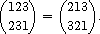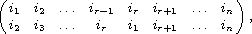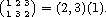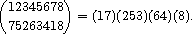Cycle decomposition

### Cycle decomposition

The points which form the first row need not be written in their natural order, e.g.Keeping this in mind, we call a permutation pÎSn a cyclic permutation or a cycle if and only if it can be written in the formwhere r ³1. In order to emphasize r we also call it an r-cycle . We note that in this case the orbits of the subgroup generated by this permutation are the following subsets of n: {i1, ...,ir }, {ir+1 }, ..., {in }. We therefore abbreviate this cycle by (i1, ...,ir)(ir+1) ...(in), where the points which are cyclically permuted are put together in round brackets. For exampleCommas which seperate the points may be omitted if no confusion can arise (e.g if n £10), and 1-cycles can be left out if it is clear which n is meant. Hence we can write p=(i1 ...ir) for the r-cycle introduced above. This cycle p can also be expressed in terms of i1 alone: p=(i1 pi1 ...pr-1i1). Using all these abbreviations and denoting by 1:=(1) ...(n) the identity element, we obtain for example

S 3= {1,(12),(13),(23),(123),(132) }.

There are the elements of the symmetric group Sn in cycle notation. The notation for a cyclic permutation is not uniquely determined, since

(i1 ...ir)=(i2 ...ir i1)= ...=(ir i1 ...ir-1).

2-cycles are called transpositions . The order of a cycle (i1 ...ir), i.e. the order of the cyclic group á(i1 ...ir) ñ generated by this cycle, is equal to its length :

| á(i1 ...ir) ñ | =r.

Two cycles p and r are called disjoint , if the two sets of points which are not fixed by p and r are disjoint sets. Notice that, for example, 1=(1)(2)(3) and (123) are disjoint cycles. Disjoint cycles p and r commute, i.e. pr= rp. (We read compositions of mappings from right to left, so that ( pr)x= p( rx).) Each permutation of a finite set can be written as a product of pairwise different disjoint cycles, e.g.There are some more examples for the cycle decomposition of a permutation.

The disjoint cyclic factors not =1 of pÎSn are uniquely determined by p and therefore we call these factors together with the fixed point cycles of p the cyclic factors of p. Let c( p) denote the number of these cyclic factors of p (including 1-cycles), let l n be their lengths, nÎ c( p) (recall that c( p)= {1, ...,c( p) }), choose, for each n an element j n of the n-th cyclic factor. Then

p= Õ nÎ c( p) (j n pj n ...pl n-1j n).

This notation becomes uniquely determined if we choose the j n so that

" m Î N : j n £pmj n, and " n<c( p) : j n<j n+1.

If this holds, then the notation as was given above is called the (standard) cycle notation for p. We note in passing that the sets {j n, pj n, ..., pl n-1j n } of points which are cyclically permuted by p are just the orbits of the cyclic group ápñ generated by p.

 harald.fripertinger "at" uni-graz.at http://www-ang.kfunigraz.ac.at/~fripert/ UNI-Graz Institut für Mathematik UNI-Bayreuth Lehrstuhl II für Mathematik
last changed: January 19, 2005Cycle decomposition